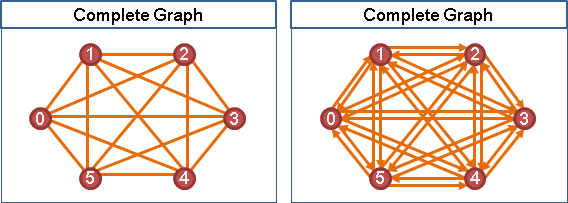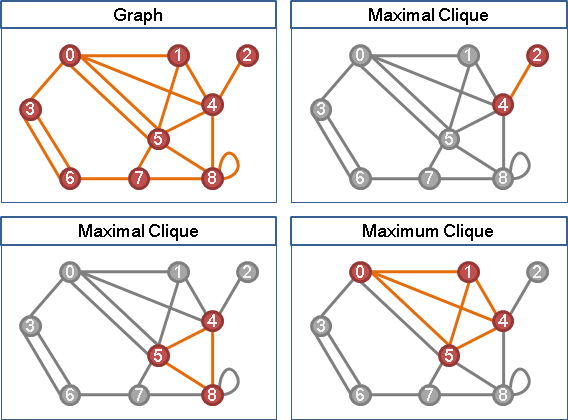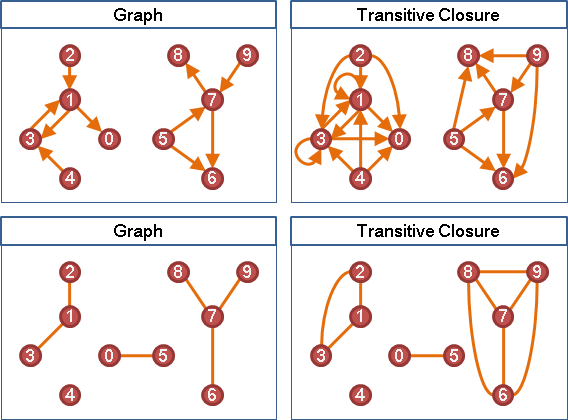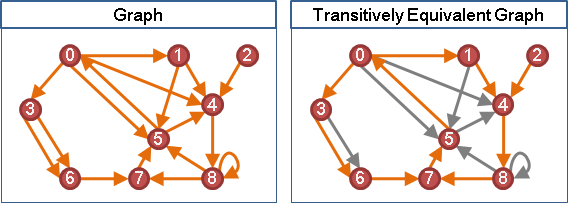Complete Graph

Complete Graph

「完全圖」。任兩點都有一條邊。Complete Subgraph（Clique）

「完全子圖」、「團」。子圖，而且任兩點都有一條邊。Minimal、Maximal是區域極值，Minimum、Maximum是全域極值。遣詞用字請小心！Maximal Clique Enumeration: Bron–Kerbosch Algorithm

Maximal Clique Enumeration

```R: 目前的clique。
P: 可以增大目前clique的點集合。接下來要列舉的點。
（與目前clique上所有點皆相鄰的點，構成的集合）
X: 可以增大目前clique的點集合，但是先前已經列舉過。用來避免重複列舉。
```

Transitive Graph

Transitive Graph

「遞移圖」。凡有路、必有邊。Transitive Subgraph

「遞移子圖」。子圖，而且凡有路、必有邊。Transitive Closure

「遞移閉包」其實是一種變換的概念，可以看做是一個函數。

「遞移閉包」。添加邊，連通關係保持相同，並且形成遞移圖。Transitively Equivalent Graph

「遞移相等圖」。子圖，而且擁有相同的遞移閉包。

「遞移相等圖」。刪除邊，連通關係保持相同。Transitive Reduction

「遞移縮減」。另外一張圖，恰好擁有相同的遞移閉包。Transitive Closure: Purdom's Algorithm

Transitive Closure: Warshall's Algorithm

Dynamic Programming。取出第k點，分成兩種情況，遞迴分割問題。

```tc(i, j, k) = tc(i, k, k-1) && tc(k, j, k-1) || tc(i, j, k-1)
^^^^^^^^^^^^^^^^^^^^^^^^^^^^^^    ^^^^^^^^^^^^^
經過第k點                         沒有經過第k點

tc(i, j, k)：由i點能不能走到j點。中繼點只能是第0點到第k點。
```

UVa 280 12017

Comparability Graph

Comparability Graph（Transitively Orientable Graph）

「可比圖」源自集合論的「偏序集Poset」，但是不盡相同。

DAG的Transitive Closure的Underlying Graph。

Mirsky's Theorem / Dilworth's Theorem

```chain：一群點，一條路徑的所有點。
anti-chain：一群點，任兩點正向不連通、逆向也不連通。
``````maximum chain length = minimum anti-chain cover
[depth]                [level]
```
```(≥) 一個level使用一條anti-chain。
(≤) 一條anti-chain不可以經過同一條chain上的點。
一條chain，每一個點至少需要一條anti-chain。
```

```minimum chain cover = maximum anti-chain length
[number of paths]     [cross all paths]
```

Greene–Kleitman Theorem

Dilworth's Theorem推廣版本。我沒有研究。

Permutation Graph

Permutation Graph

```(G == permutation) iff (G == comparability && ~G == comparability)
if (G == permutation) then (~G == permutation)
```

ICPC 6508